### THE KONGNER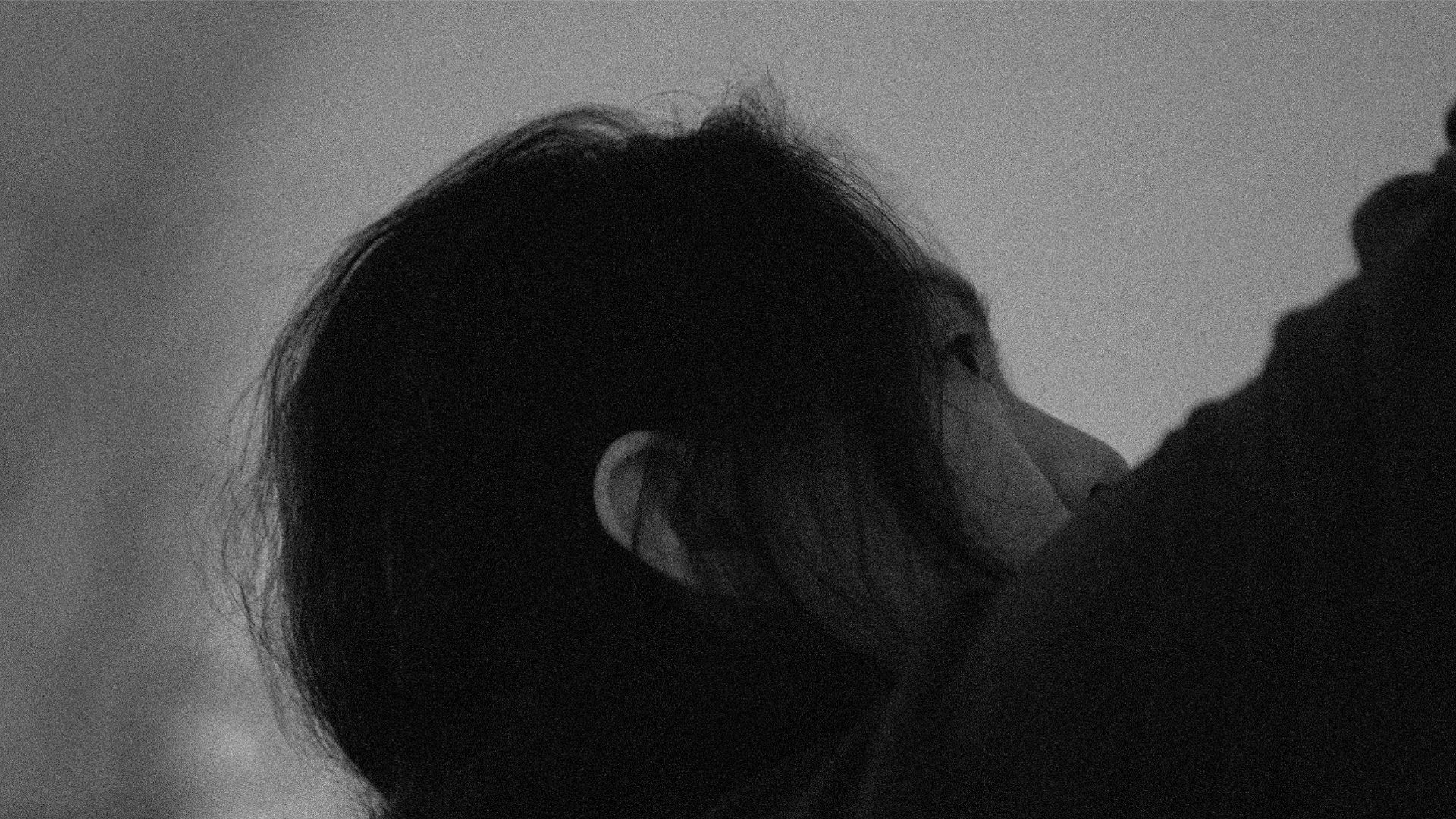• MUSIC COMPOSER
• DIALOGUE 01

# Every Noiseis Poetic withOlivier Cong江逸天：聲音皆是詩意

• Dialogue 01
• -
• Olivier Cong
• Music Composer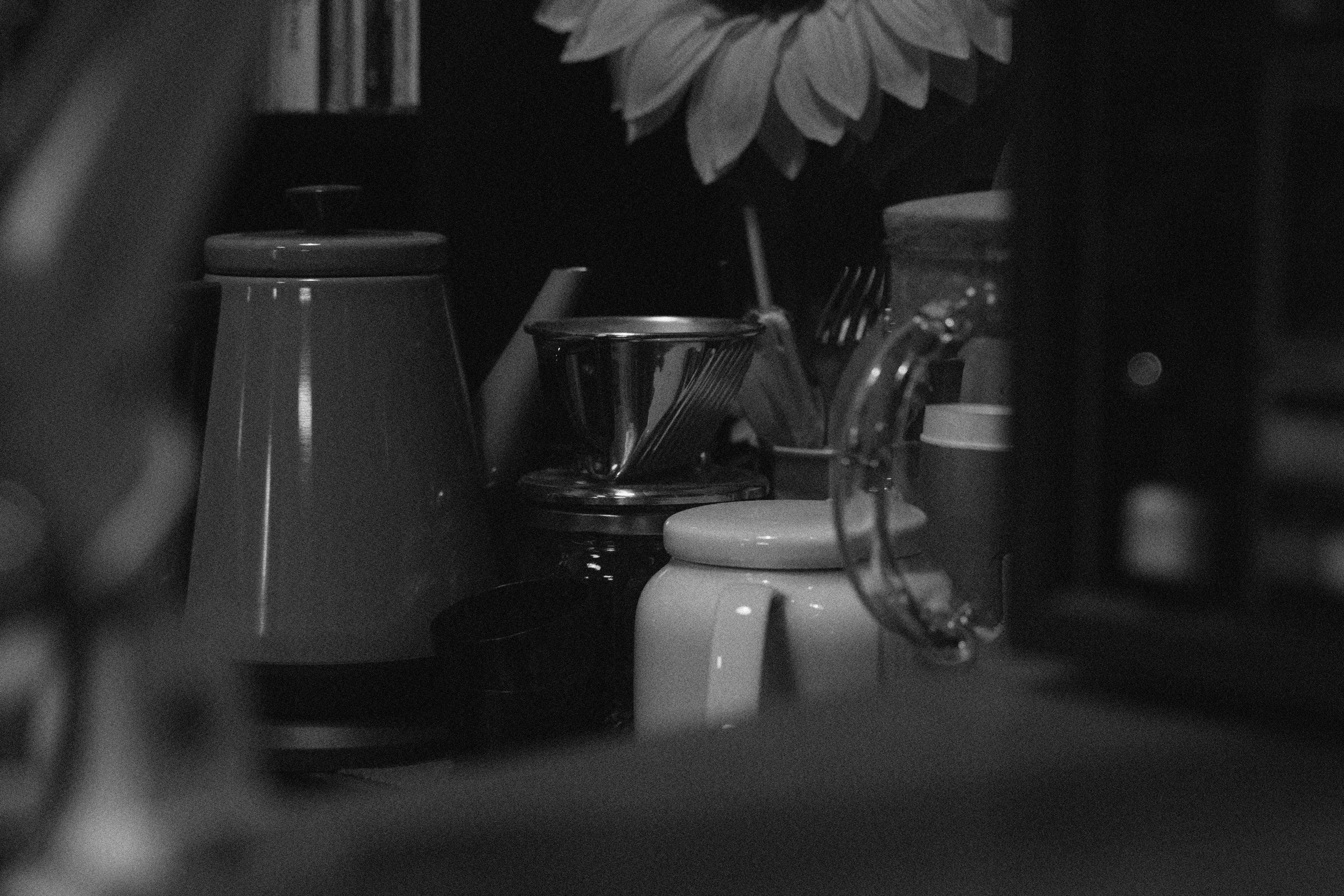K

O

K

O

K

O

K

O

K

O

K

O

K

O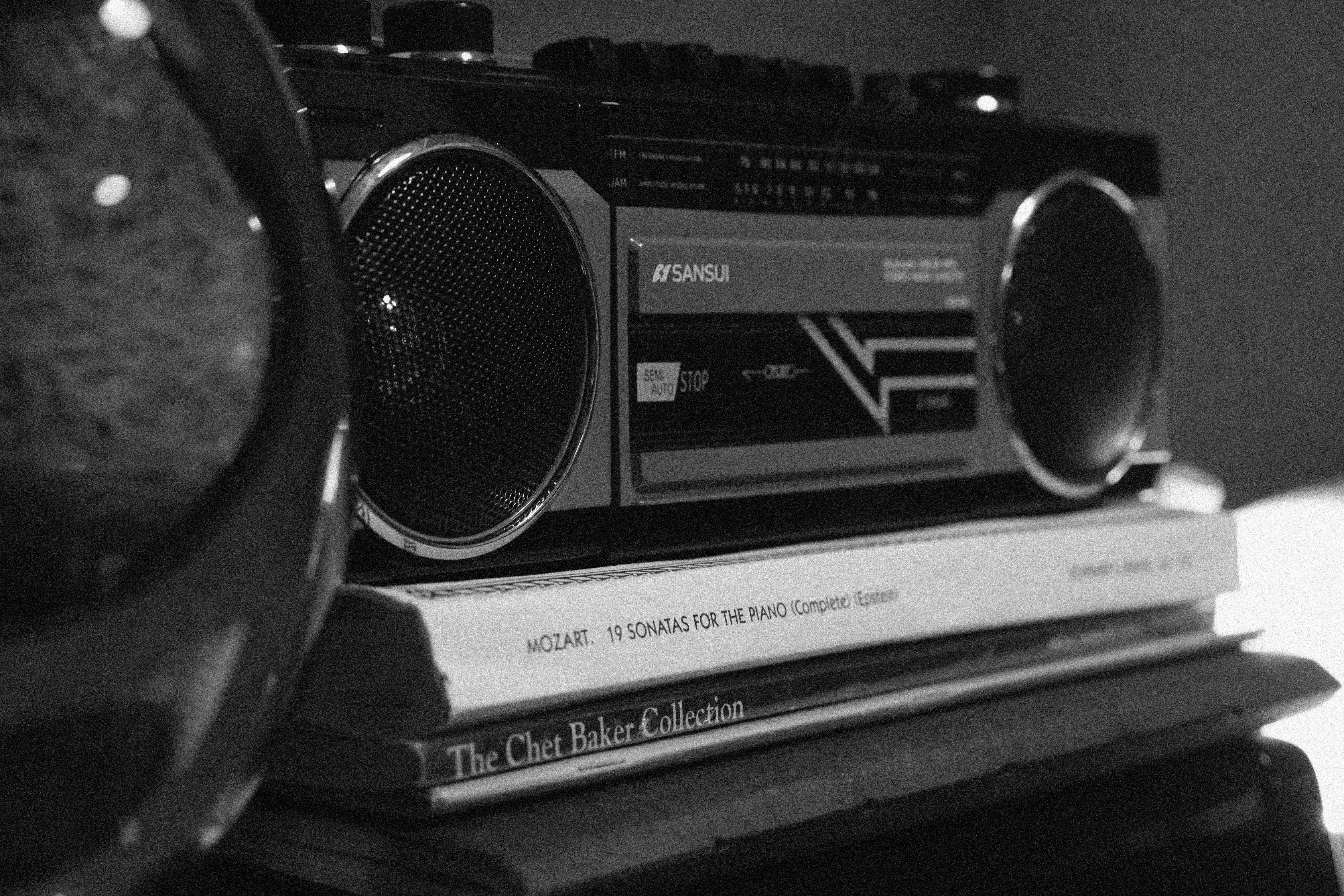K

O

K

O

K

O

K

O

K

O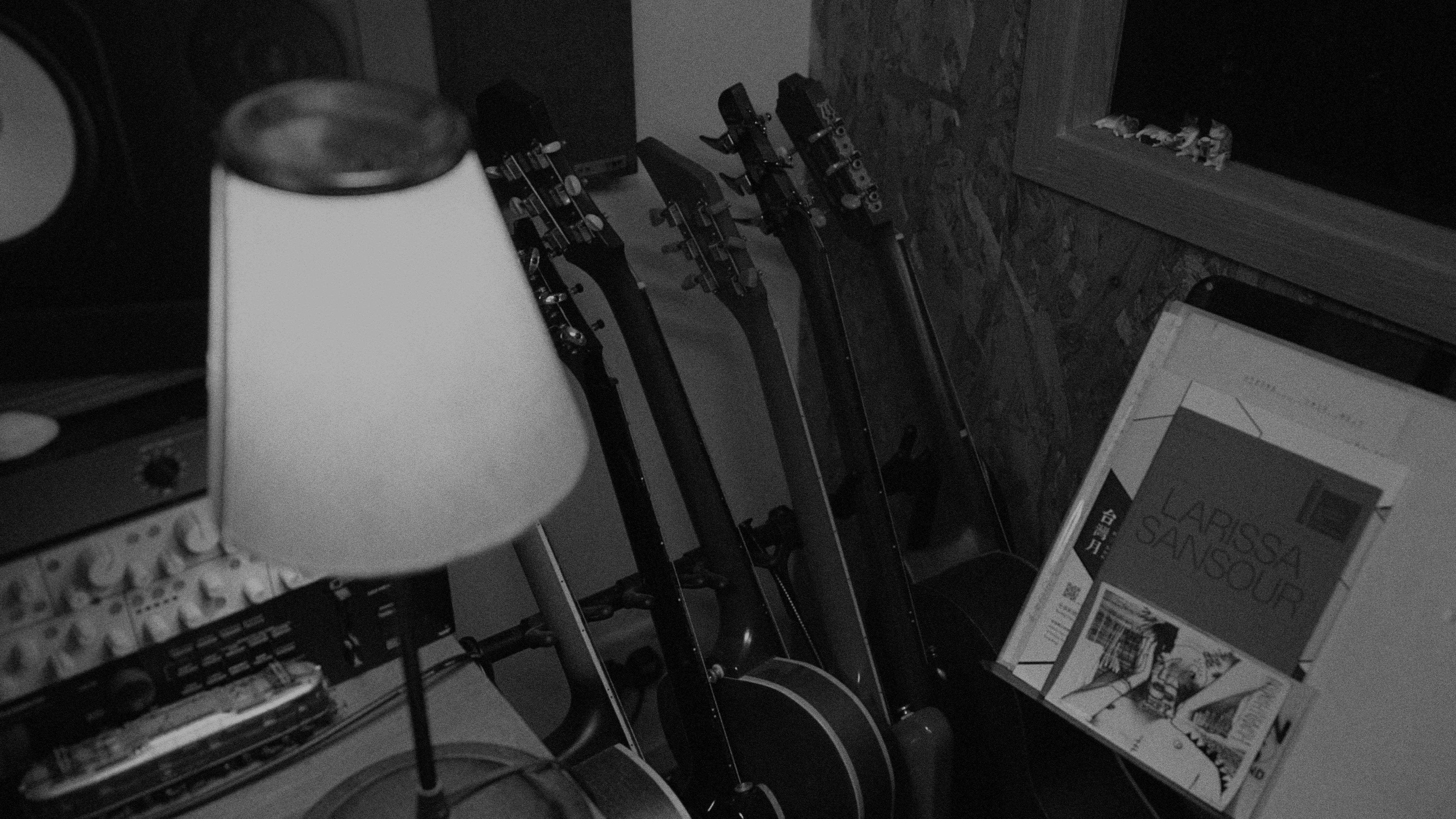K

O

K

O

K

O

K

O

K

O

K

O

K

O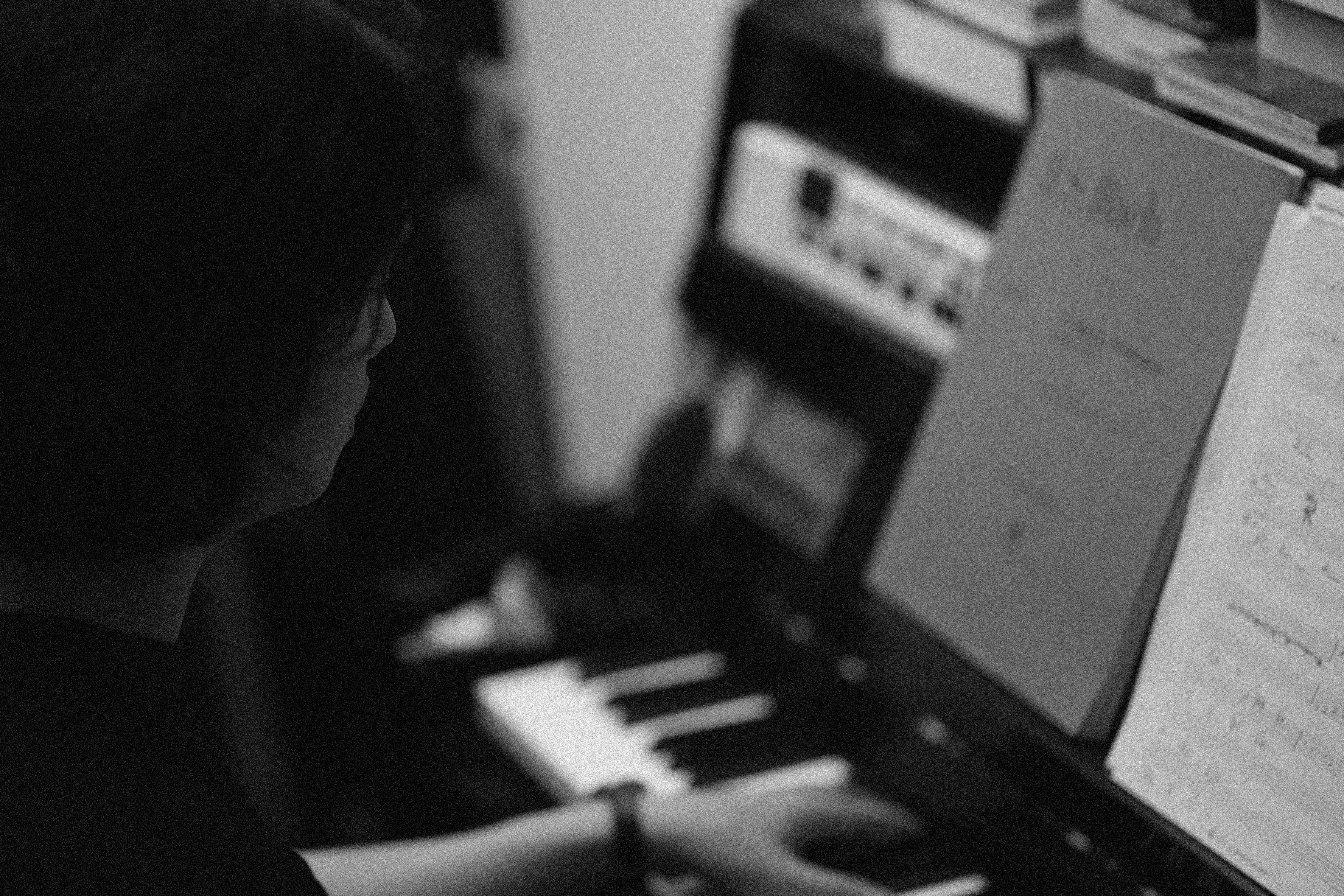K

O

K

O

K

O

K

（笑）那你還喜歡吃些什麼呢？

O

K

O

K

O

K

O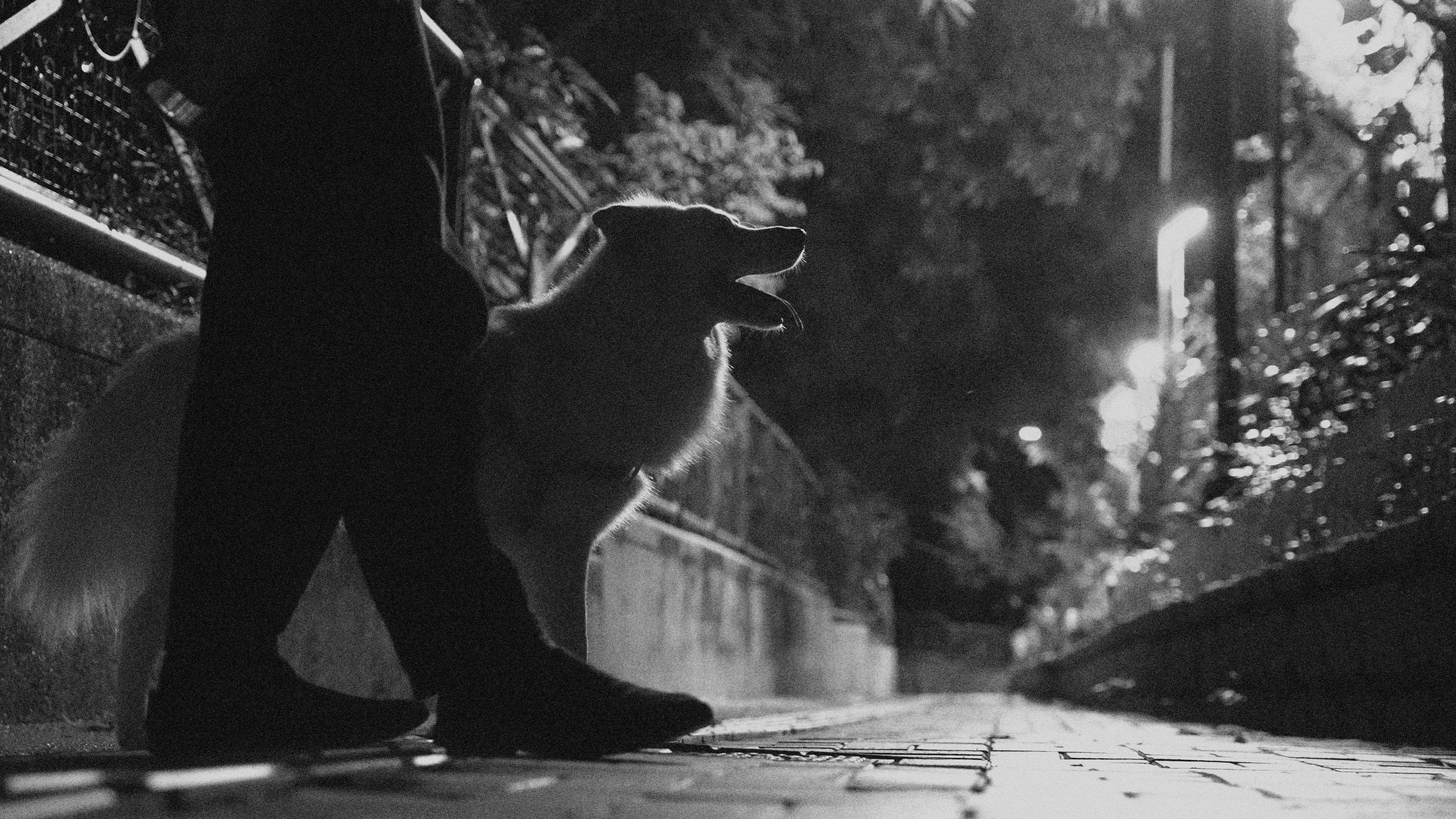K

O

K

O

K

O

K

O

K

O

K

O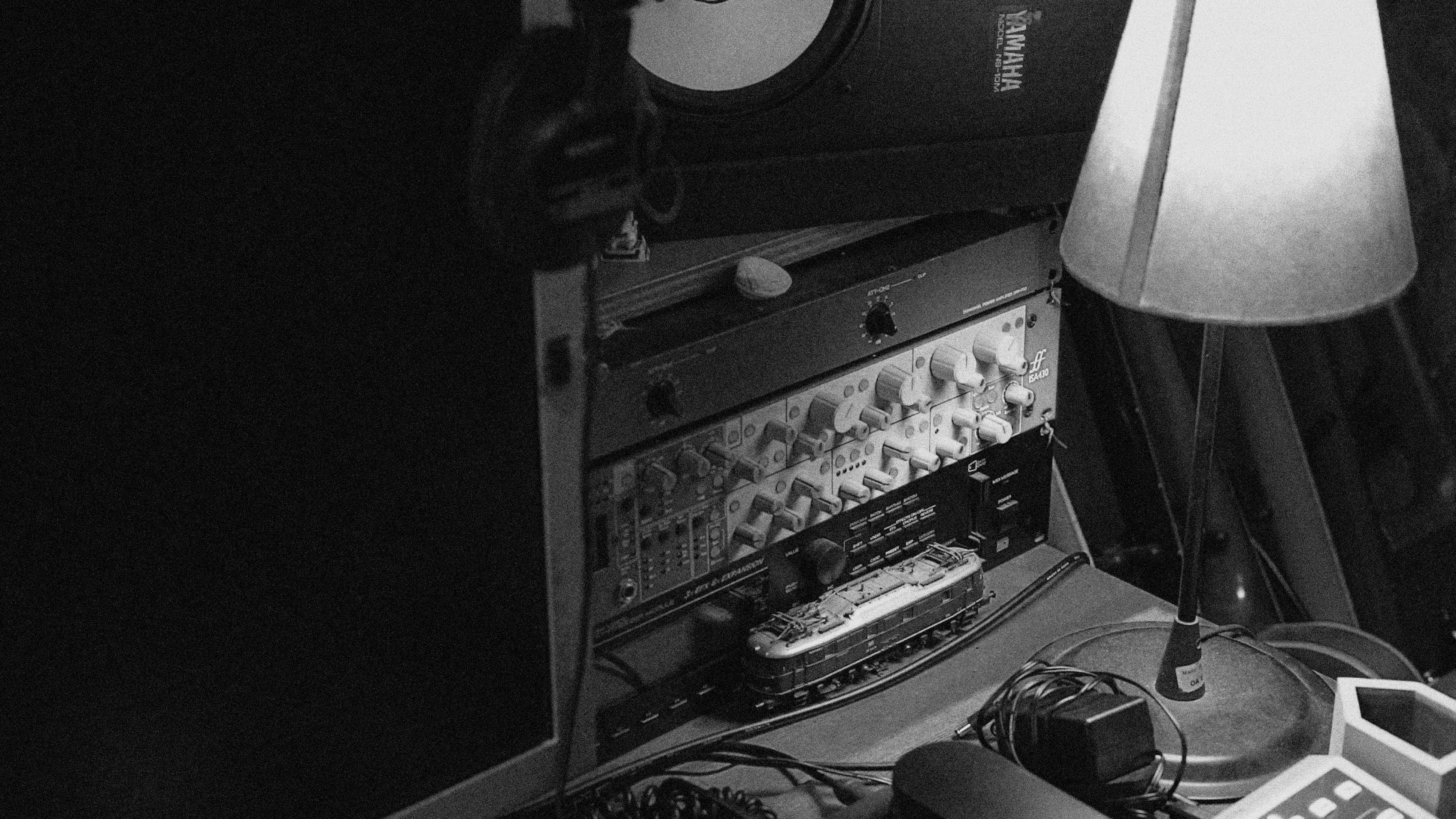K

O

K

O

K

O

K

O

K

O

K

O

K

O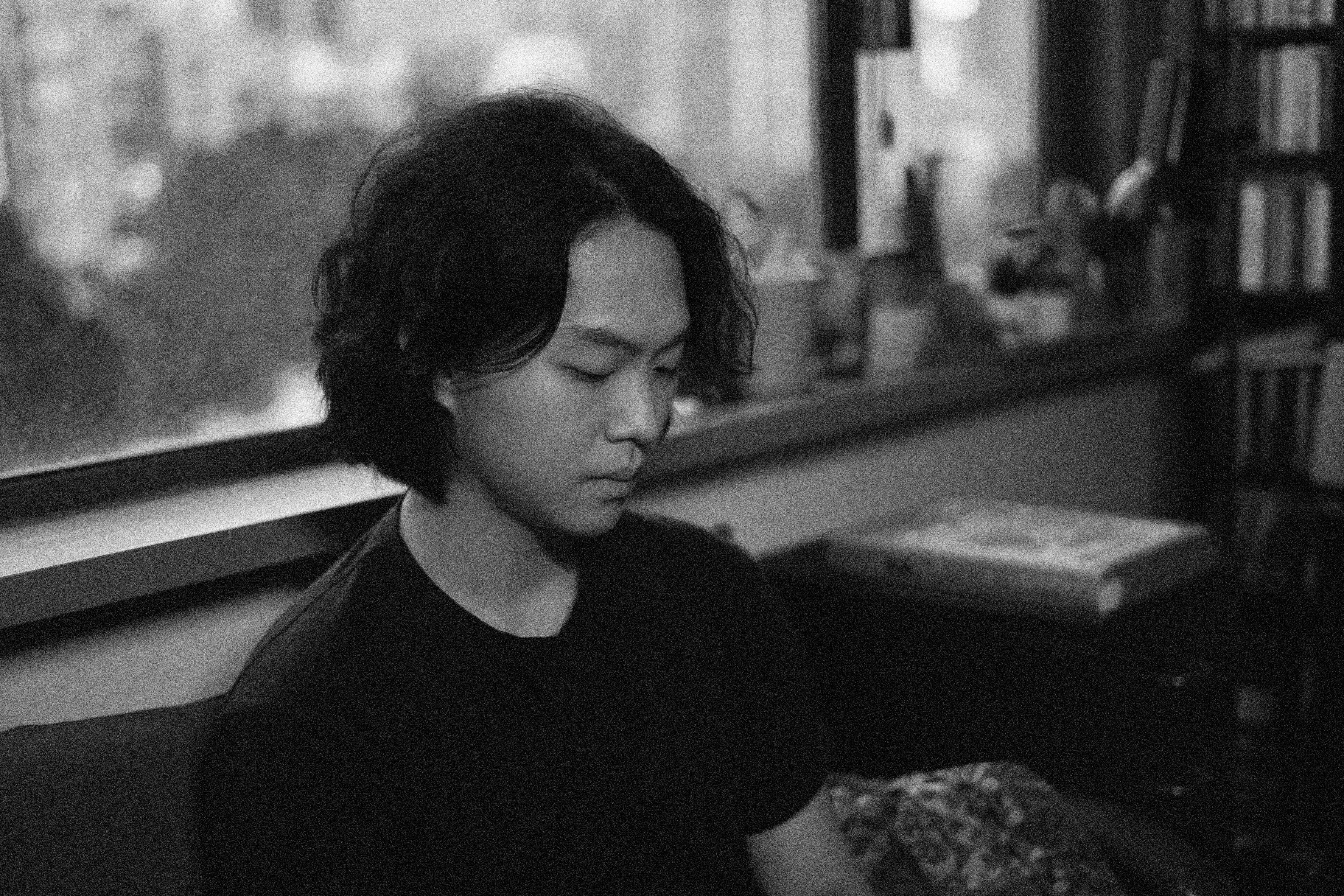K

O

K

O

K

O

K

O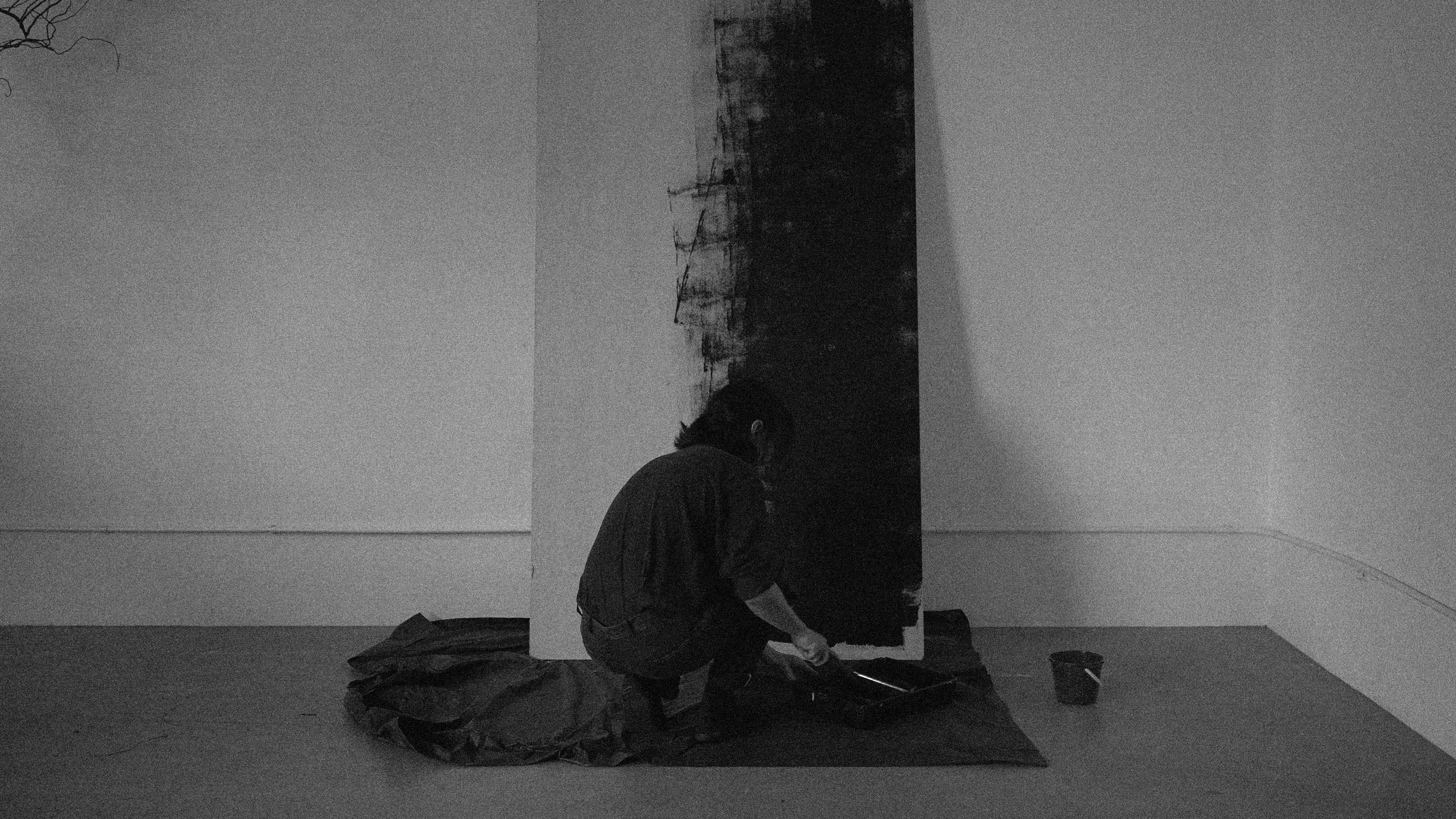K

O

K

O

K

O

K

O

K

O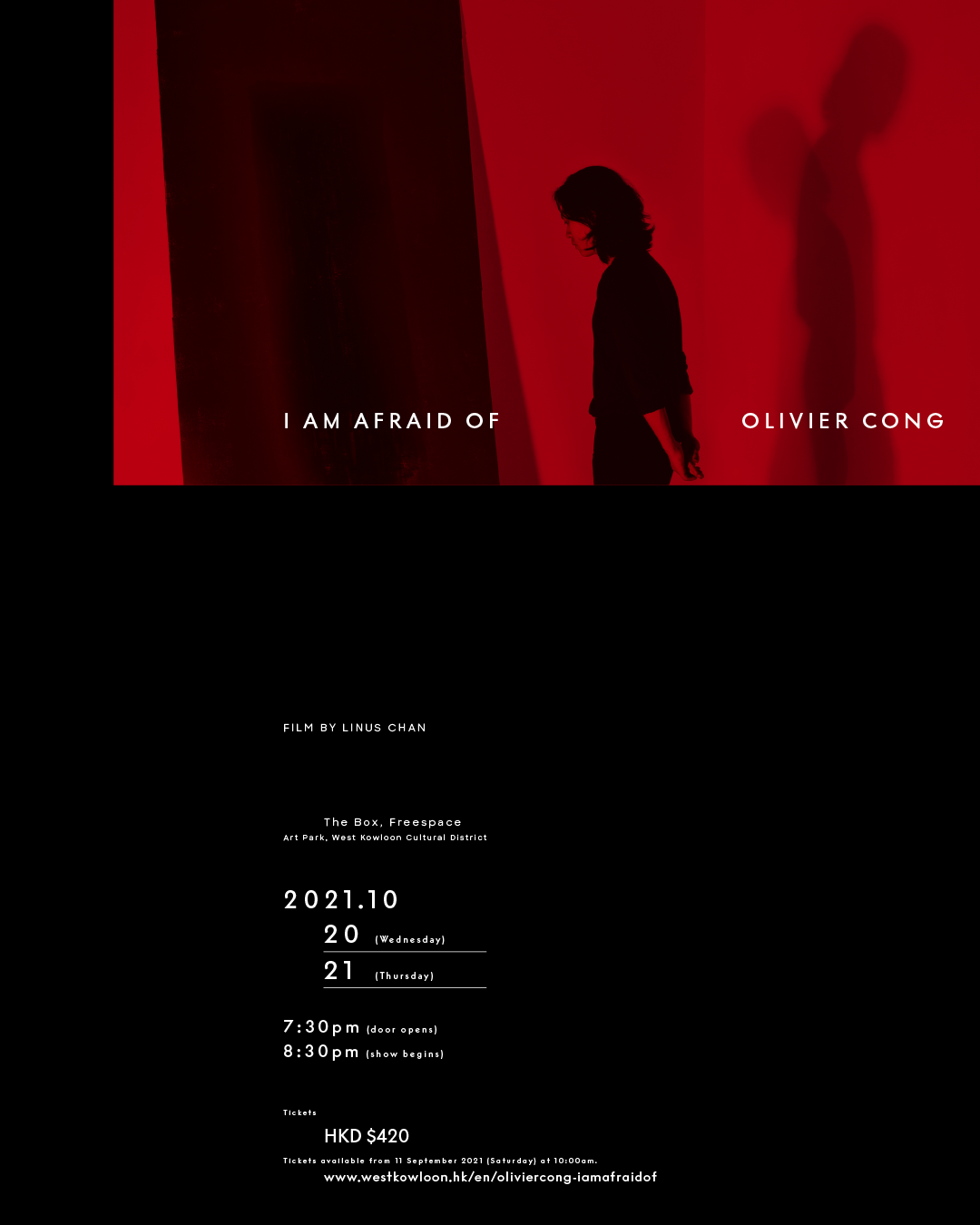K

O

K

O

K

O

K

O